This section briefly describes a problem for which the HJB equation can be directly solved to yield a closed-form expression, as opposed to an algorithm that computes numerical approximations. Suppose that a linear system is given by (13.37), which requires specifying the matrices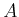and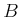. The task is to design a feedback plan that asymptotically stabilizes the system from any initial state. This is an infinite-horizon problem, and no termination action is applied.

An optimal solution is requested with respect to a cost functional based on matrix quadratic forms. Let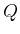be a nonnegative definite15.4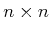matrix, and let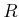be a positive definitematrix. The quadratic cost functional is defined as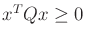(15.20)

To guarantee that a solution exists that yields finite cost, several assumptions must be made on the matrices. The pair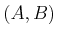must be stabilizable, and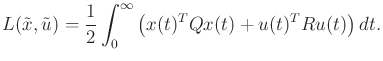must be detectable; see  for specific conditions and a full derivation of the solution presented here.

Although it is not done here, the HJB equation can be used to derive the algebraic Riccati equation,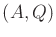(15.21)

in which all matrices except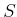were already given. Methods exist that solve for, which is a unique solution in the space of nonnegative definitematrices.

The linear vector field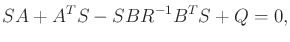(15.22)

is asymptotically stable (the real parts of all eigenvalues of the matrix are negative). This vector field is obtained ifis selected using a feedback plan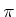defined as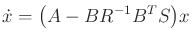(15.23)

The feedback planis in fact optimal, and the optimal cost-to-go is simply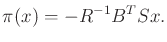(15.24)

Thus, for linear systems with quadratic cost, an elegant solution exists without resorting to numerical approximations. Unfortunately, the solution techniques do not generalize to nonlinear systems or linear systems among obstacles. Hence, the planning methods of Chapter 14 are justified.

However, many variations and extensions of the solutions given here do exist, but only for other problems that are expressed as linear systems with quadratic cost. In every case, some variant of Riccati equations is obtained by application of the HJB equation. Solutions to time-varying systems are derived in . If there is Gaussian uncertainty in predictability, then the linear-quadratic Gaussian (LQG) problem is obtained . Linear-quadratic problems and solutions even exist for differential games of the form (13.204) .

Steven M LaValle 2012-04-20